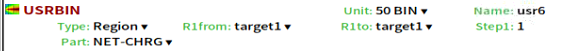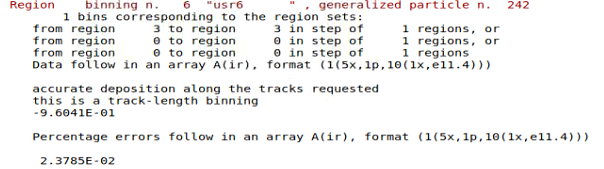# Normalization of USRBIN NET-CHRG results

Dear experts,
I am running a FLUKA simulation with an electron beam hitting an aluminum plate (called tagert1).

I’m interested in the total net charge of target1, so I use USRBIN to score it and get the result as following.However,the simulation is run with a certain number of primary electrons(15000 in my case).There is a parameter called beam current in electron accelators,often in the unit of mA.

I learn from the Internet that 1mA means there are 6.241E15 electrons crossing a certain section per second.

I want to simulate 1mA beam current of electron accerlator,but the number of electorns is too large for the card START to set.

Therefore,I have two questions:
(1) If I multiply the USRBIN result by 1.602E-19(elementary electron charge)and 6.241E15(beam intensity),can it reflect the real situation?

(2) If not,how can I set an electron beam source containing such a large number of electrons versus time?(As 1mA means 6.241E15 electrons per second, I’d like to set the source for ten seconds)

Dear @shiqiang.pang ,

The number specified in the START card is the number of independent histories ( i.e. primary particles ) that FLUKA will simulate in order to estimate the requested quantity. In your case (and in most cases), the result provided by FLUKA is already normalized per primary particle so the number specified in that card will not affect your normalization. In addition to this, the NET-CHRG is provided in units of elementary electron charge.

Therefore, to obtain the total net charge deposited in target1 per second by a 1 mA electron accelerator you should multiply the result of your USRBIN by 6.2415E15 ( primary particles per second ) to get it in units of elementary charge. You will need to multiply it also by 1.6022E-19 if you want the result in Coulomb.

In short, yes to your first questionHope it helps.

Kind regards,
Francisco

1 Like

A post was split to a new topic: Normalization of USRBIN DOSE results